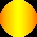ON SOME REGULAR TOROIDS

LAJOS SZILASSI

 Name: Lajos Szilassi (b. Szentes, Hungary, 1942). Address:Department of Mathematics, Juhász Gyula Teachers Training College, University of Szeged, Boldogasszony str 6, Szeged, H-6701 Hungary; E-mail: szilassi@jgytf.u-szeged.hu Fields of interest:Geometry (polyhedra), Computer graphics, (programming, visualization). Publications: Szilassi, L. (1986) Regular toroids, Structural Topology, [Montreal], No. 13, pp. 69-80.

 Abstract: As it is known, in a regular polyhedron every face has the same number of edges and every vertex has the same number of edges, as well. A polyhedron is called topologically regular if further conditions (e.g. on the angle of the faces or the edges) are not imposed. An ordinary polyhedron is called a toroid if it is topologically torus-like (i.e. it can be converted to a torus by continuous deformation), and its faces are simple polygons. A toroid is said to be regular if it is topologically regular. It is easy to see, that the regular toroids can be classified into three classes, according to the number of edges of a vertex and of a face. There are infinitely many regular toroids in each class, because the number of the faces and vertices can be arbitrarily large. Hence, we study mainly those regular toroids, whose number of faces or vertices is minimal, or that ones, which have any other special properties. Among these polyhedra, we take special attention to the so called Császár-polyhedron", which has no diagonal, i.e. each pair of vertices are neighbouring, and its dual polyhedron (in topological sense) the so called Szilassi-polyhedron", whose each pair of faces are neighbouring. The first one was found by Ákos Császár in 1949, and the latter one was found by theauthor, in 1977.

1 ON THREE CLASSES OF TOROIDS

For simple (sphere-like) polyhedra, Euler's formula V - E + F = 2 holds, where V, E and F are the numbers of vertices, edges and faces, respectively. For toroids Euler's formula is modified to V - E + F = 0. Studying toroids, we cannot expect all of the faces or solid angles to be regular and congruent, so the description regular here is clearly a topological property.

Assume each face of a regular toroid has a edges, and at each vertex exactly b edges meet. We can distinguish three classes of regular toroids, according to the numbers of edges incident with each face and each vertex: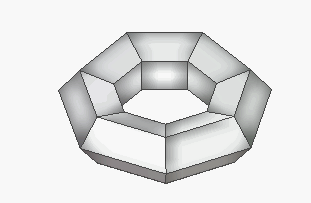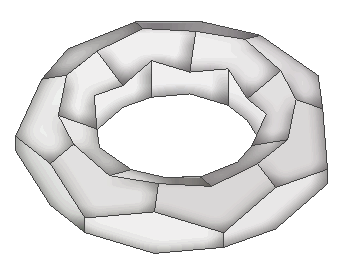Figure 1:
class T1 : a=3, b=6; class T2: a=4, b=4; class T3,: a=6, b=3;

However, it is interesting to determine for each class the lowest number of faces or vertices required to construct a regular toroid in that class, possibly with the restricting condition that the faces or solid angles belong to as few congruence classes as possible.

2 THE CSÁSZÁR POLYHEDRON

At every vertex of a regular toroid in class T1 exactly six edges meet, so that such a toroid has at least seven vertices.

The Császár polyhedron , ,  (pp. 244-246) is such a toroid with only seven vertices. This polyhedron does indeed belong to class T1 for any two of its vertices are joined by an edge, and thus six edges meet at each vertex. The number of its vertices is the lowest possible not only in class T1 , it can readily be seen that a toroid with less than seven vertices does not exist. The toroid, which is constructed on the basis of the data published by Professor Ákos Császár at Budapest University who is a member of the Hungarian Academy of Sciences , appears "fairly crowded". We have prepared a computer program to search for a "less crowded" version.

Without going into the details a formal definition (which would need oriented matroid terms ), we only restrict ourselves to an intuitive description as follows. Let us consider two models of Császár polyhedron essentially congruent if:

• one of the polyhedra can be transferred into the other by gradually changing the coordinates of one of the polyhedra without causing self intersection in the surface;
• one of the polyhedra can be transferred into the other by reflection;
• one of the polyhedra can be transferred into the other by executing the above operations one after another;
otherwise the two Császár-polyhedra are essentially different.

J. Bokowski and A. Eggert proved in 1986 that the Császár polyhedron has only four essentially different versions.

It is to be noted that in topological terms the various versions of Császár polyhedron are isomorphic, there is only one way to draw the full graph with seven vertices on the torus.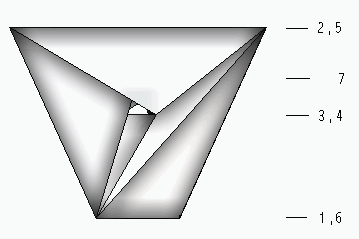Figure 2. The full graph with seven vertices that can be drawn on the torus

3 POLYHEDRA FROM CLASS T2

Class T2 of regular toroids consists of those torus-like ordinary polyhedra in which four edges meet at each vertex and the faces are quadrilaterals. (Figure 3.) This type of regular toroids is the easiest to construct.Figure 3. Two toroids with 35 faces, and one with min (9) faces in class T2

It is not known whether there exists a regular toroid in T2 with 10 or 11 faces (vertices), though a graph having 10 or 11 vertices (and regions), and which belongs to T2, class can be drawn on torus.

4 SOME POLYHEDRA FROM CLASS T3

When constructing toroids belonging to class T3, care should be taken to make sure that the six points defining one region (face) are in the same plane. This requirement can be easily met, if the toroid has "sufficiently high number" of faces. (Figure 1.) We have just created some interesting regular toroid consisting of 24, 9 and of 7 faces only (Figure 4).

As we have seen, the most important property of the Császár polyhedron is that any two vertices are joined by an edge. There is a very close relationship, so-called duality, between this polyhedron and the polyhedron with the lowest number of faces in class T3, the main characteristic of the latter being that any two faces have a common edge This relationship is partly topological, however, if we create a new polyhedron by using projective transformation from a given polyhedron, (e.g. polarity with respect to a sphere), then the new polyhedron may be a self-intersecting one.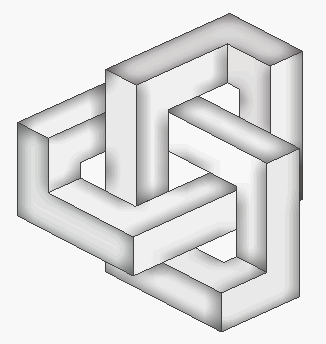Figure 4. Toroids from class T3 with 24, 9 and 7 faces

The author of this paper constructed this seven-faced polyhedron in 1977 after producing the dual of the Császár polyhedron by using polarity. The new polyhedron obtained by this way, had self-intersecting faces, too. A computer assisted analysis had used to find the undesired intersections, and by modifying the data could be obtained the above polyhedron bordered by simple polygons. (Figure 4.) If a model of the structure is known, it is easy to develop a simple method to construct the polyhedron. This polyhedron is topologically isomorphic with Heawood's toroidal map with seven colours. (It was Martin Gardner who used the term "Szilassi polyhedron" for the first time to identify this polyhedron .) In 2004 J. Bokowski and L. Schewe has proved that the Szilassi polyhedron had essentially only one axially symmetric version .

References

 Bokowski, J. and Eggert, A. (1991) All realizations of Möbius' torus with 7 vertices, Structural Topology, No. 17, pp. 59-78.

 Bokowski, J. and Scewe, L. (2004) On Szilassis Torus, Preprint Technishe Hochschule Darmstadt.

 Császár, Á. (1949) A polyhedron without diagonals, Acta Sci. Math. Universitatis Szegediensis, 13, 140-142.

 Gardner, M. (1978) The minimal art , Scientific American, 1978/11.

 Gardner, M. (1992) Fractal Music, Hypercards, and More Mathematical Recreations from Scientific American Magazine, New York: W. H. Freeman, 118-120.

 Gruber, P.M. and Wills, J.M. (1993) Handbook of Convex Geometry, Amsterdam: North-Holland.

 Grünbaum, F. (1994) Polytopes, Kluwer Academic Publishers.

 Kappraff, J. (2001) Connections: The Geometric Bridge between Art and Science, Singapore: World Scientific.

 Szilassi, L. (1986) Regular toroids, Structural Topology, No. 13, 69-80.

 Stewart, B. M. (1980) Adventures Among the Toroids, Revised 2nd edition, Okemos, Michigan.